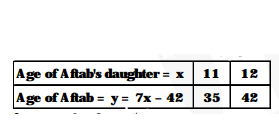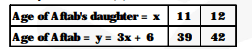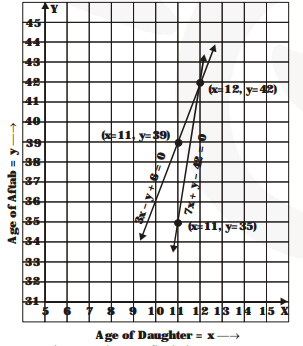# Aftab tells his daughter, "Seven years ago, I was seven times as old as you were thenQuestion.

Aftab tells his daughter, "Seven years ago, I was seven times as old as you were then. Also, three years from now, I shall be three times as old as you will be". (Isn't this interesting?) Represent this situation algebraically and graphically.

Solution:

Let the present age of Aftab's daughter = x years.

and the present age of $A f t a b=y$ years $(y>x)$

According to the given conditions

Seven years ago,

$(y-7)=7 \times(x-7)$

i.e., $\quad y-7=7 x-49$

i.e., $\quad 7 x-y-42=0$...(1)

Three years later, $(y+3)=3 \times(x+3)$

i.e., $\quad y+3=3 x+9$

i.e., $\quad 3 x-y+6=0$ ...(2)

Thus, the algebraic relations are $7 x-y-42=0,3 x-y+6=0$.

Now, we represent the problem graphically as below : $7 x-y-42=0$ ...(1)$3 x-y+6=0 \quad$...(ii)From the graph, we find that

x = 12

and y = 42

Thus, the present age of Aftab's daugther = 12 years

and the present age of Aftab = 42 years